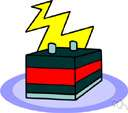# volt

(redirected from Petavolt)
Also found in: Thesaurus, Medical, Encyclopedia.

## volt 1

(vōlt)
n. Abbr. V
The SI-derived unit of electric potential and electromotive force, equal to the difference of electric potential between two points on a conducting wire carrying a constant current of one ampere when the power dissipated between the points is one watt. See Table at measurement.

[After Count Alessandro Volta.]

## volt 2

also volte  (vōlt, vôlt)
n. Sports
1. A circular movement executed by a horse in manège.
2. A sudden movement made in avoiding a thrust in fencing.

[French volte, from Italian volta, turn, from voltare, to turn, leap; see vault2.]

## volt

(vəʊlt)
n
(Units) the derived SI unit of electric potential; the potential difference between two points on a conductor carrying a current of 1 ampere, when the power dissipated between these points is 1 watt. Symbol: V
[C19: named after Count Alessandro Volta2]

(vɒlt) or

## volte

n
1. (Horse Training, Riding & Manège) a small circle of determined size executed in dressage
2. (Fencing) a leap made in fencing to avoid an opponent's thrust
[C17: from French volte, from Italian volta a turn, ultimately from Latin volvere to turn]
Collins English Dictionary – Complete and Unabridged, 12th Edition 2014 © HarperCollins Publishers 1991, 1994, 1998, 2000, 2003, 2006, 2007, 2009, 2011, 2014

## volt1

(voʊlt)

n.
the SI unit of potential difference and electromotive force, equal to the difference of electric potential between two points of a conductor carrying a constant current of one ampere, when the power dissipated between these points is equal to one watt. Abbr.: V
[1870–75; after A. Volta]

## volt2

(voʊlt)

n.
1. a circular movement or gait in manège in which a horse going sideways turns around a center with its head facing outward.
2. a sudden leap in fencing to avoid a thrust.
[1650–60; < French volte < Italian volta, n. derivative of voltare to turn < Vulgar Latin *volvitare]

## volt

(vōlt)
A unit used to measure electromotive force. One volt is equal to the force that carries one ampere of current through a conductor that has a resistance of one ohm.

## volt

1. The unit of potential difference.
2. (V) A unit of electromotive force and potential difference, equal to the difference in potential between two points of a conducting wire carrying a constant current of one ampere (A), when the power released between the points is one watt (W). Named after the Italian physicist Alessandro Volta (1745–1827).
Dictionary of Unfamiliar Words by Diagram Group Copyright © 2008 by Diagram Visual Information Limited
ThesaurusAntonymsRelated WordsSynonymsLegend:
 Noun 1volt - a unit of potential equal to the potential difference between two points on a conductor carrying a current of 1 ampere when the power dissipated between the two points is 1 watt; equivalent to the potential difference across a resistance of 1 ohm when 1 ampere of current flows through itVpotential unit - a measure of the potential energy of a unit charge at a given point in a circuit relative to a reference point (ground)abvolt - a unit of potential equal to one-hundred-millionth of a voltmillivolt, mV - a unit of potential equal to one thousandth of a voltmicrovolt - a unit of potential equal to one millionth of a voltnanovolt - a unit of potential equal to one billionth of a voltpicovolt - a unit of potential equal to one trillionth of a voltfemtovolt - a unit of potential equal to one quadrillionth of a volt (or one thousandth of a nanosecond)kilovolt, kV - a unit of potential equal to a thousand volts
Based on WordNet 3.0, Farlex clipart collection. © 2003-2012 Princeton University, Farlex Inc.
Translations
فُولْتفولط: وِحْدَة القُوَّة الكَهْرُبائِيَّه
волт
volt
volt
volt
voltti
volt
volt
volt
ボルト
볼트
voltas
volts
volt
volt
volt
โวลต์หน่วยแรงดันไฟฟ้า
вольт
vôn

## volt

[vəʊlt] N
Collins Spanish Dictionary - Complete and Unabridged 8th Edition 2005 © William Collins Sons & Co. Ltd. 1971, 1988 © HarperCollins Publishers 1992, 1993, 1996, 1997, 2000, 2003, 2005

## volt

[ˈvəʊlt] nvolt m
Collins English/French Electronic Resource. © HarperCollins Publishers 2005

## volt

nVolt nt
Collins German Dictionary – Complete and Unabridged 7th Edition 2005. © William Collins Sons & Co. Ltd. 1980 © HarperCollins Publishers 1991, 1997, 1999, 2004, 2005, 2007

## volt

[vəʊlt] nvolt m inv
Collins Italian Dictionary 1st Edition © HarperCollins Publishers 1995

## volt

(vəult) noun
(often abbreviated to V) the unit used in measuring the force driving electricity through a circuit, or the strength of an electric current.
ˈvoltage (-tidʒ) noun
(a) force measured in volts. Low voltage reduces the current, making the lights burn dimly.
Kernerman English Multilingual Dictionary © 2006-2013 K Dictionaries Ltd.

## volt

volt volt voltti volt ボルト 볼트 volt หน่วยแรงดันไฟฟ้า vôn
Multilingual Translator © HarperCollins Publishers 2009

n voltio